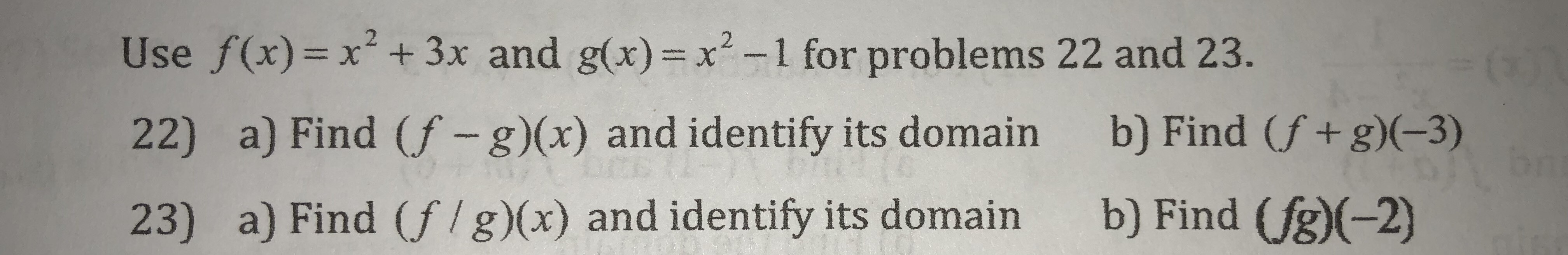Use /x)- x + 3x and gop)- x' -1 for problems 2 and 23.22) a) Find (f -g)(x) and identify its domain23) a) Find (f/g)(x) and identify its domainb) Find (f +g)(-3)b) Find (jg)

Questionhelp_outlineImage TranscriptioncloseUse /x)- x + 3x and gop)- x' -1 for problems 2 and 23. 22) a) Find (f -g)(x) and identify its domain 23) a) Find (f/g)(x) and identify its domain b) Find (f +g)(-3) b) Find (jg) fullscreen
Step 1

The operation (f+g)(x) means f(x) + g(x), the operation (f-g)(x) means f(x) - g(x). Domain means the values of x which gives a defined output to the function.We ne...

Want to see the full answer?

See Solution

Want to see this answer and more?

Our solutions are written by experts, many with advanced degrees, and available 24/7

See Solution
Tagged in

Algebra